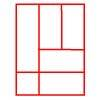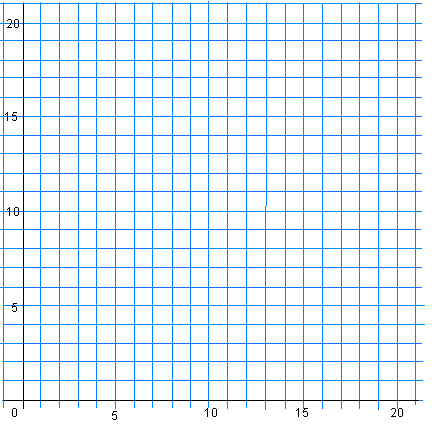#### You may also like### Bracelets

Investigate the different shaped bracelets you could make from 18 different spherical beads. How do they compare if you use 24 beads?### Cut and Make

Cut a square of paper into three pieces as shown. Now,can you use the 3 pieces to make a large triangle, a parallelogram and the square again?### Is a Square a Rectangle?

How many rectangles can you find in this shape? Which ones are differently sized and which are 'similar'?

# A Cartesian Puzzle

##### Age 7 to 11 Challenge Level:

Here are the coordinates of some quadrilaterals, but in each case one coordinate is missing!  The coordinates are given going round each quadrilateral in an anti-clockwise direction.

1. $(2,11), \; (0,9),\; (2,7),\; (?,?)$
2. $(3,7),\; (3,4),\; (8,4),\; (?,?)$
3. $(18,3),\; (16,5), \;(12,5),\; (?,?)$
4. $(13,12),\; (15,14),\; (12,17),\; (?,?)$
5. $(7,14),\; (6,11),\; (7,8),\; (?,?)$
6. $(15,9),\; (19,9),\; (16,11),\; (?,?)$
7. $(11,3),\; (15,2),\; (16,6),\; (?,?)$
8. $(9,16),\; (2,9),\; (9,2),\; (?,?)$

The quadrilaterals are all symmetrical. This may be rotational or line symmetry or both. Can you work out what the missing coordinates are if you know they are all positive? Is there more than one way to find out?

Now plot those eight missing coordinates on a graph like this. What shape do they make and what sort of symmetry does it have?{Please note that this activity was slightly adjusted on the 18th June 2015, thanks to a comment we received}